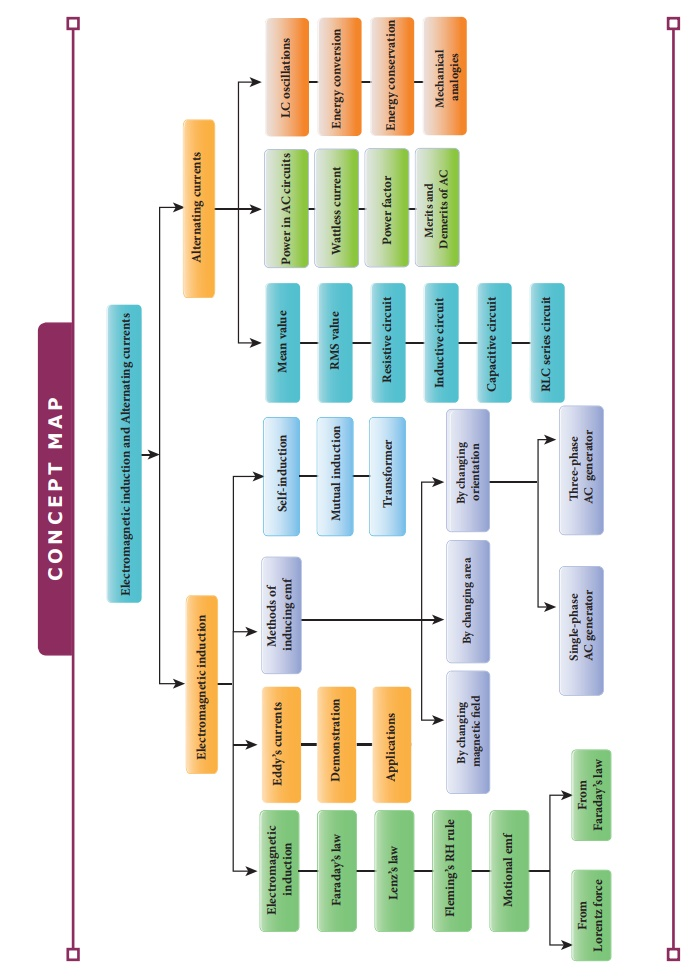Home | | Physics 12th Std | Electromagnetic Induction and Alternating Current: Summary

# Electromagnetic Induction and Alternating Current: Summary

Whenever the magnetic flux linked with a closed coil changes, an emf is induced and hence an electric current flows in the circuit. This phenomenon is known as electromagnetic induction.

SUMMARY

Â·        Whenever the magnetic flux linked with a closed coil changes, an emf is induced and hence an electric current flows in the circuit. This phenomenon is known as electromagnetic induction.

Â·        Faradayâ€™s first law states that whenever magnetic flux linked with a closed circuit changes, an emf is induced in the circuit.

Â·        Faradayâ€™s second law states that the magnitude of induced emf in a closed circuit is equal to the time rate of change of magnetic flux linked with the circuit.

Â·        Lenzâ€™s law states that the direction of the induced current is such that it always opposes the cause responsible for its production.

Â·        Lenzâ€™s law is established on the basis of the law of conservation of energy.

Â·        Flemingâ€™s right hand rule states that if the index finger points the direction of the magnetic field and the thumb indicates the direction of motion of the conductor, then the middle finger will indicate the direction of the induced current.

Â·        Even for a conductor in the form of a sheet or a plate, an emf is induced when magnetic flux linked with it changes. The induced currents flow in concentric circular paths called Eddy currents or Foucault currents.

Â·        Inductor is a device used to store energy in a magnetic field when an electric current flows through it.

Â·        If the flux linked with the coil is changed, an emf is induced in that same coil. This phenomenon is known as self-induction. The emf induced is called self-induced emf.

Â·        When an electric current passing through a coil changes with time, an emf is induced in the neighbouring coil. This phenomenon is known as mutual induction and the emf is called mutually induced emf.

Â·        AC generator or alternator is an energy conversion device. It converts mechanical energy used to rotate the coil or field magnet into electrical energy.

Â·        In some AC generators, there are three separate coils, which would give three separate emfs. Hence they are called three-phase AC generators.

Â·        Transformer is a stationary device used to transform AC electric power from one circuit to another without changing its frequency.

Â·        The efficiency of a transformer is defined as the ratio of the useful output power to the input power.

Â·        An alternating voltage is a voltage which changes polarity at regular intervals of time and the resulting alternating current changes direction accordingly.

Â·        The average value of alternating current is defined as the average of all values of current over a positive half-circle or negative half-circle.

Â·        The root mean square value or effective value of an alternating current is defined as the square root of the mean of the squares of all currents over one cycle.

Â·        A sinusoidal alternating voltage (or current) can be represented by a vector which rotates about the origin in anti-clockwise direction at a constant angular velocity. Such a rotating vector is called a phasor.

Â·        When the frequency of the applied alternating source is equal to the natural frequency of the RLC circuit, the current in the circuit reaches its maximum value. Then the circuit is said to be in electrical resonance.

Â·        The magnification of voltages at series resonance is termed as Qâ€“factor.

Â·        Power of a circuit is defined as the rate of consumption of electric energy in that circuit. It depends on the components of the circuit.

Â·        Whenever energy is given to a LC circuit, the electrical oscillations of definite frequency are generated. These oscillations are called LC oscillations.

Â·        During LC oscillations, the total energy remains constant. It means that LC oscillations take place in accordance with the law of conservation of energy.Study Material, Lecturing Notes, Assignment, Reference, Wiki description explanation, brief detail
12th Physics : Electromagnetic Induction and Alternating Current : Electromagnetic Induction and Alternating Current: Summary |